# Reformer - The Efficient Transformer

### Backgroud

REFORMER: THE EFFICIENT TRANSFORMER

1. Transformer架構無法採用long-sequence，最多只能採用 512的token來作為句子訓練，並且batch_size依據實驗也不適合過大。
2. TransformerSelf-Attention的機制當中，是每一個Query要與其他token的Key去做Dot-Product，因此會造成時間複雜度 O(N^2)，即便句子很短也需要這麼多的時間。
3. TransformerEncoder架構當中有一個sublayer-FFN，其內部的hidden-size比input, output size來得大，這會造成許多GPU記憶體的浪費。
4. Transformer會存每一個layer activation後的結果，會造成記憶體用量N被成長。
然而，Reformer為了解決這些痛點提出了幾個解決方針，且促使整體的時間複雜度從 O(N^2)降到 O(NlogN)，其中提出的方法列舉如下:
1. Locality Sensitive Hashing(LSH) Attention
2. Reversible layers
3. Chunking FFN layers


### Locality Sensitive Hashing(LSH) Attention

\begin{equation}
Attention(Q,K,V) = softmax(\frac{QK^T}{\sqrt{d_k}})V
\end{equation}

7 mod 3 = 2
4 mod 3 = 1
2 mod 3 = 2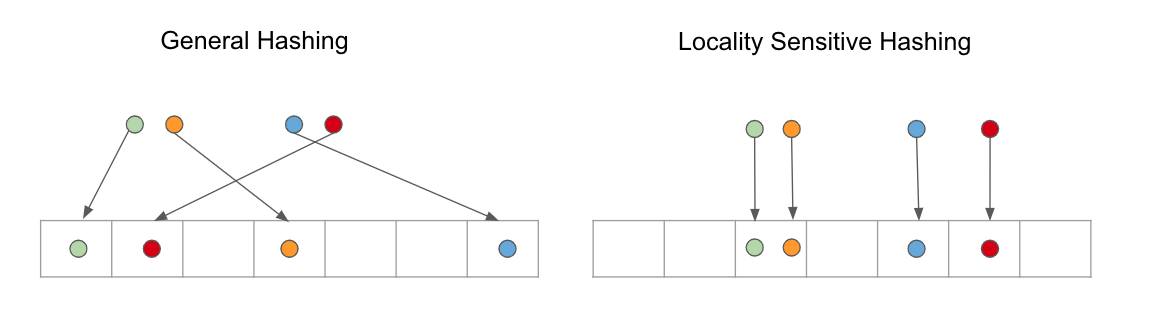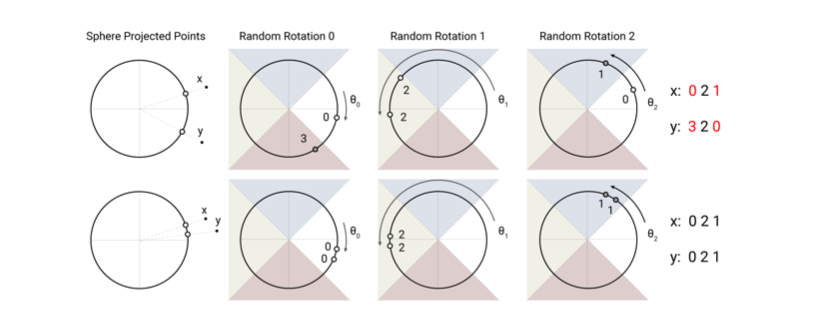\begin{equation}
oi = \sum{j \in{P_i}} exp(q_i \cdot k_j-z(i, P_i))v_j
\end{equation}

\begin{equation}
P_i = j: h(q_i) = h(k_l)
\end{equation}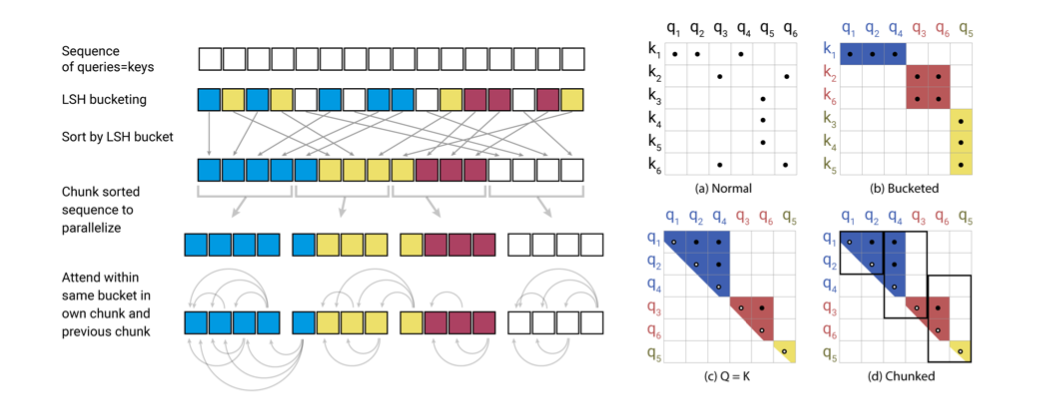• 圖(a)這邊可以看到這是 Encoder的Attention，因為正常情況下$q_3$是無法關注到$k_6$，這邊可以看到對於要關注的位置是稀疏
• 圖(b)接著透過 LSH來關注到相同 Bucket內的 keys，並且按照Bucket來做排序，因此會得到圖(b)。我們可以發現一個Bucket中會有多的Query但很少Key的情況。
• 所以這時候做Shard-QK Attention，也就是將Q=k，來解決Q,K不均衡的問題。而圖(C)也可以看到有『空心』的符號，因為在Shard-QK Attention如果關注自己本身會造成其他的attention score很低，因此必須加以排除。
• 圖(d)最後，即便Q=K，會出現有個Bucket個數很多，有些Bucket個數很少，然而也會有Bucket佔據了一些Keys，為了讓LSH有效果，作者在這邊加入了Chunk機制，會先設定每個Bucket的大小 $m=\frac{2l}{n_{bucket}}\qquad$，($l$為query的個數)，對於每一個Bucket來說可以關注自己當前與前一個Bucket中相同Hash的Key因此 $\tilde{P_i}$ 轉換如下:

\begin{equation}
oi = \sum_{j \in{P_i}} exp(q_i \cdot k_j-z(i, P_i))v_j
\end{equation}

1. 先設定Q=K
2. 執行 LSH Bucket，來歸類到每一個Bucket中
3. 依據bucket來做排序
4. 進行Chunk的拆分
5. 最後每一個Bucket的Query可以關注當前與前一個bucket相同的Key


#### Multi-round LSH attention### Reverible Transformer

Transformer當中的sublayer，每次的attention會與FFN做連結，就必須記憶每一個layer的activation，這會造成記憶體浪費，這裡作者引用了RevNet的作法，不保留中間層的activation，之記住最後一層的activation，接著透過推導來求得每一層的輸入。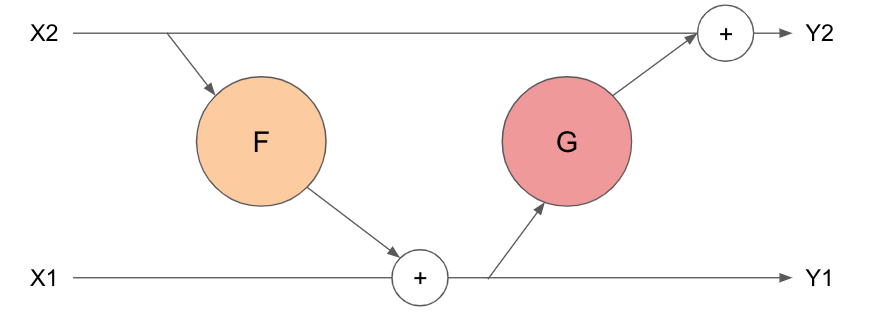\begin{equation}
y_1 = x_1 + F(x_2)\
y_2 = x_2 + G(y_1)
\end{equation}

Reverible的倒傳遞圖如下: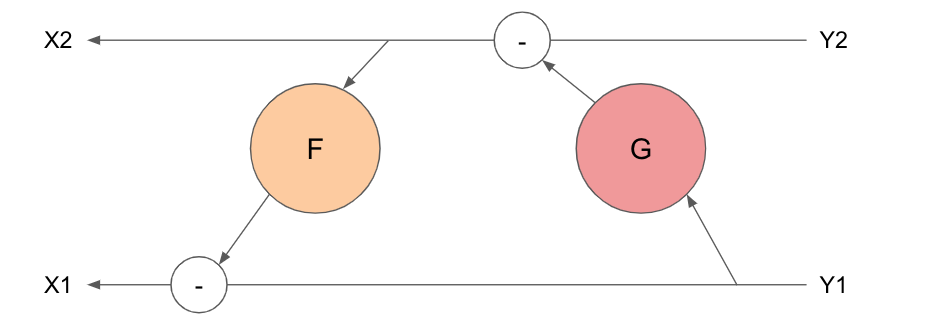\begin{equation}
x_2 = y_2 - G(y_1)\
x_1 = y_1 - F(x_2)
\end{equation}

• 可以看到計算 $x_2$時只運用了上一層的 activation值 $y_1$, $y_2$，而計算$x_1$則是運用前一步進算出來的$x_1$，因此不需要儲存額外的activation(時間換空間)
• 透過這樣節省記憶體空間，Reformer可計算更長的sequence。

\begin{equation}
y_1 = x_1 + Attention(x_2)
y_2 = x_2 + FFN(y_1)
\end{equation}

### Chunking

\begin{equation}
Y_2 = [Y_2^{(1)}; ...; Y_2^{(c)}] = [X_2^{(1)} + FeedForward(Y_1^{(1)});...;X_2^{(c)} + FeedForward(Y_1^{(c)})]
\end{equation}

### Experiments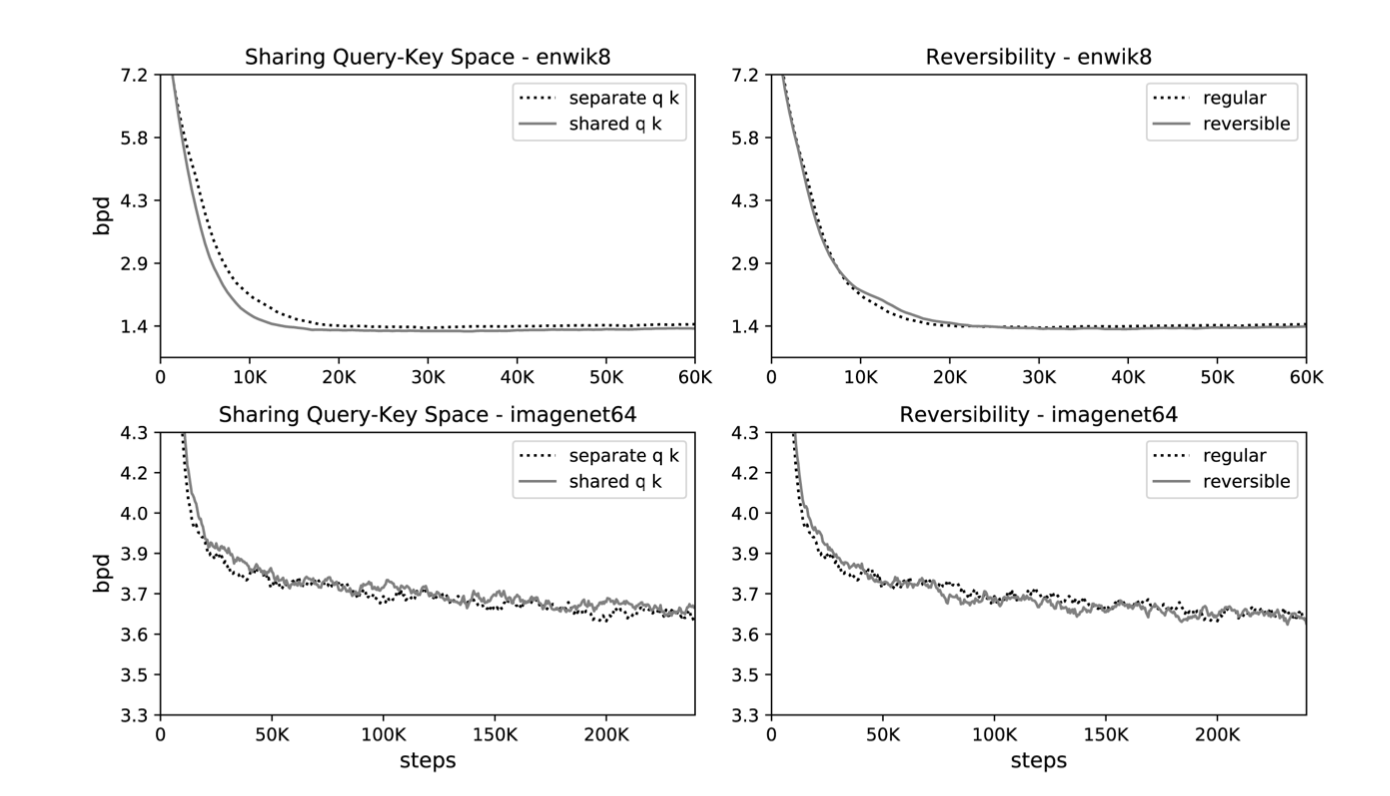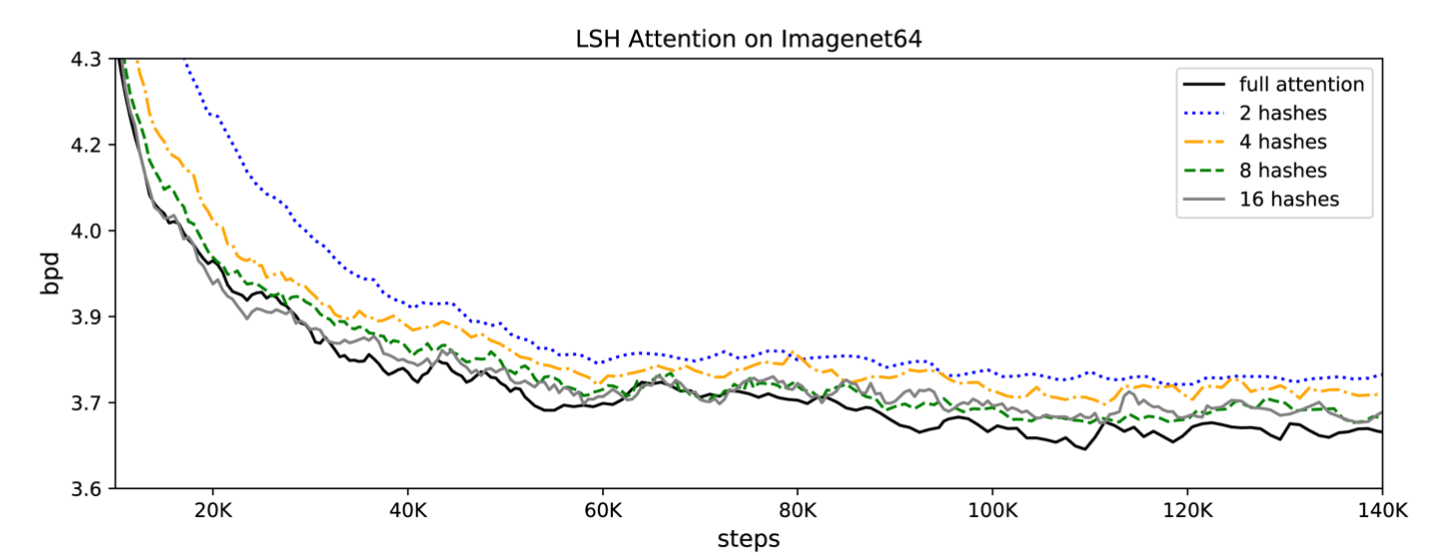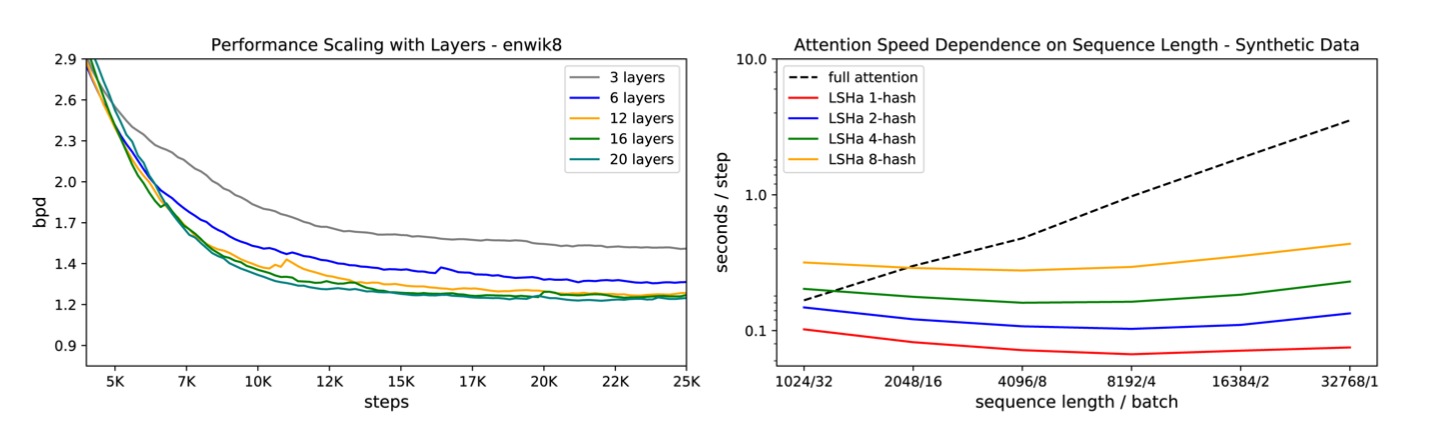### Conclusion

Reformer除了延伸了Transformer的精神之外，也改善了一些缺點，包含使用較少的記憶體訓練與計算、可訓練較長的Sequece、也可以在單機做訓練等，有了這些改進，就可以對於較長的句子、文本可以作更好的訓練效果，也可以應用到其他的領域來做長sequence訓練且提升訓練速度與效益。